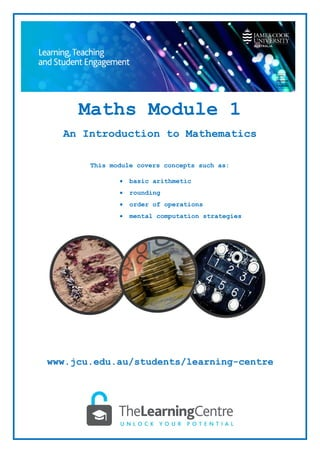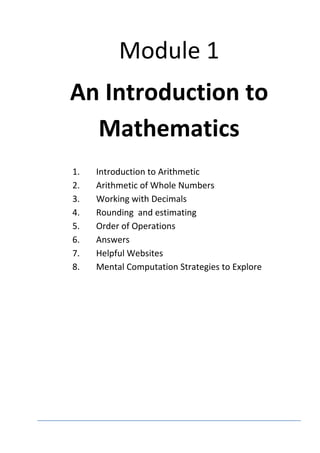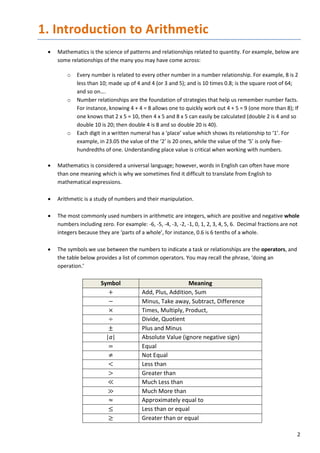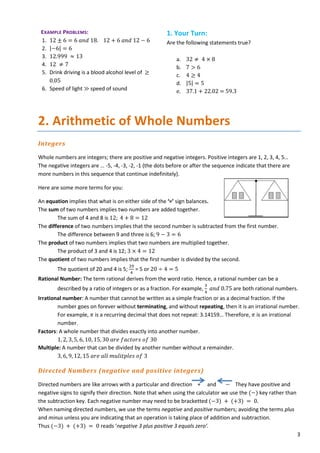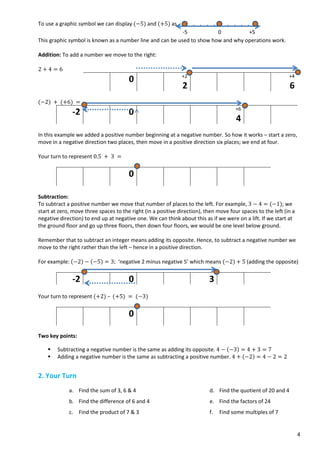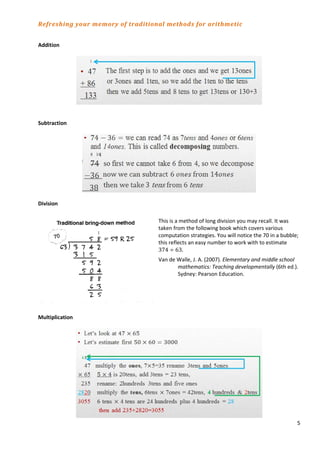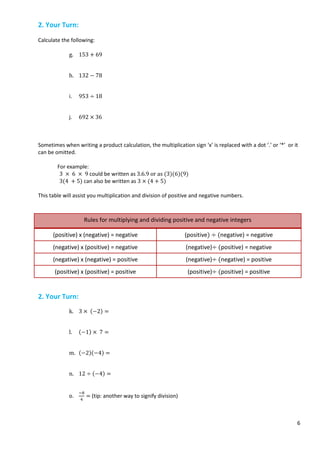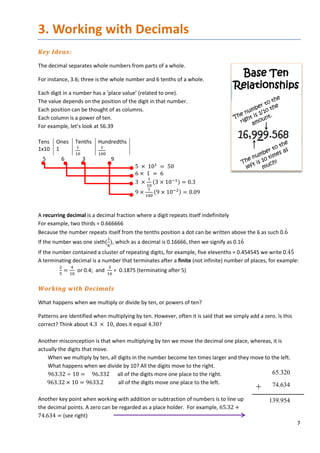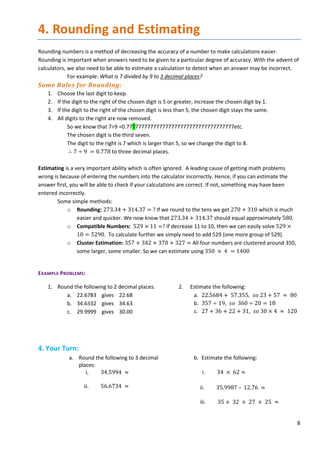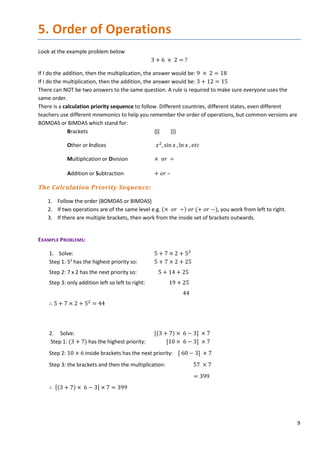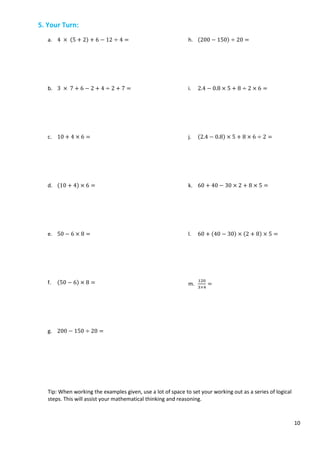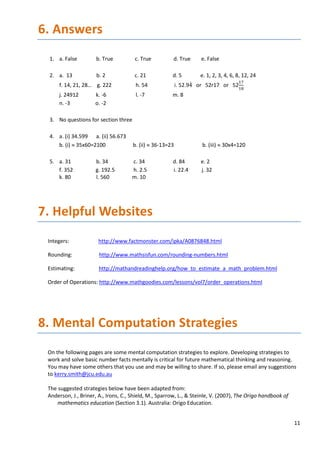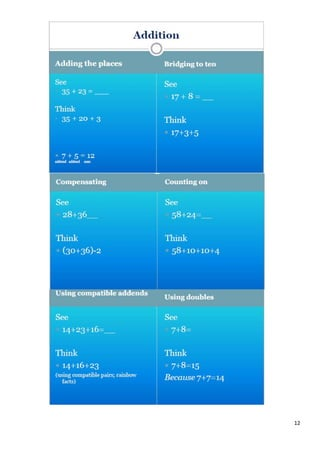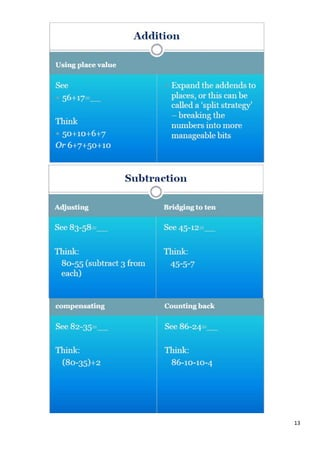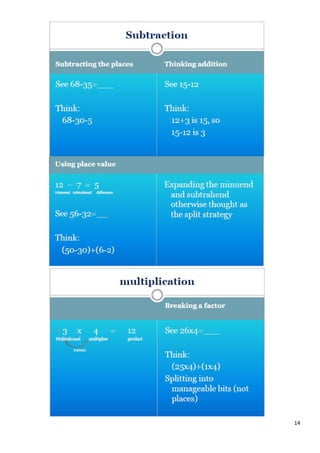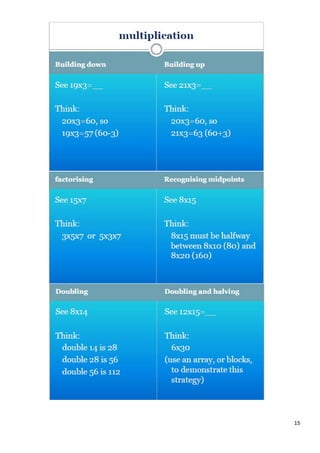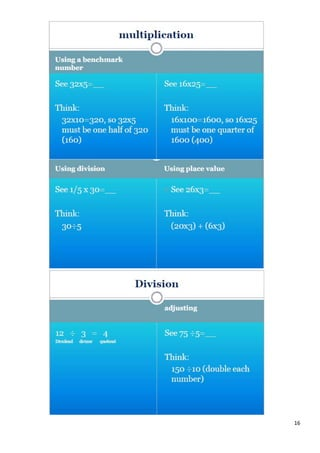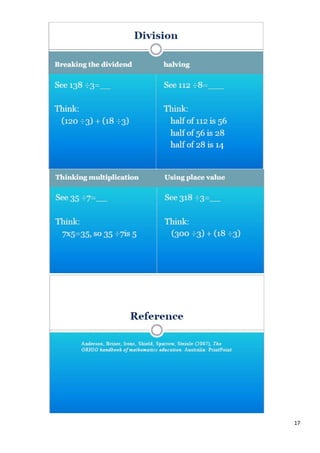Nächste SlideShare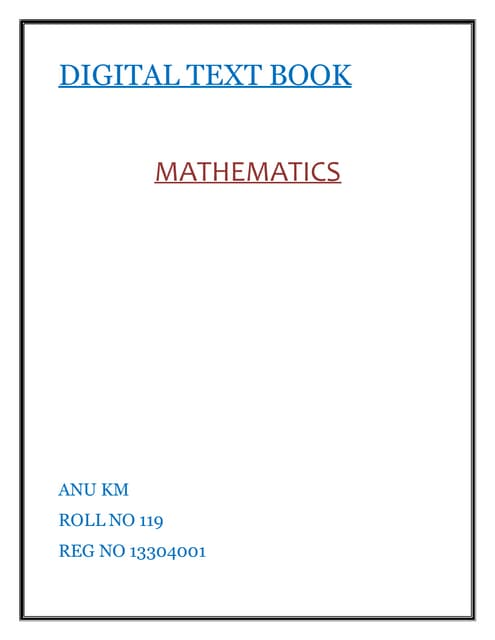Digital textbook
1 von 18Digital textbookanumolkmWHOLE NUMBERS (5).pptxSphesihle18Marh algebra lessonM, Michelle JeanniteBasic math conceptsPRINTDESK by DanSecondary LectureAmalia Indrawati Gunawan

### 1-Introduction-to-Maths.pdf

1. Maths Module 1 An Introduction to Mathematics This module covers concepts such as: • basic arithmetic • rounding • order of operations • mental computation strategies www.jcu.edu.au/students/learning-centre
2. Module 1 An Introduction to Mathematics 1. Introduction to Arithmetic 2. Arithmetic of Whole Numbers 3. Working with Decimals 4. Rounding and estimating 5. Order of Operations 6. Answers 7. Helpful Websites 8. Mental Computation Strategies to Explore
3. 2 1. Introduction to Arithmetic • Mathematics is the science of patterns and relationships related to quantity. For example, below are some relationships of the many you may have come across: o Every number is related to every other number in a number relationship. For example, 8 is 2 less than 10; made up of 4 and 4 (or 3 and 5); and is 10 times 0.8; is the square root of 64; and so on…. o Number relationships are the foundation of strategies that help us remember number facts. For instance, knowing 4 + 4 = 8 allows one to quickly work out 4 + 5 = 9 (one more than 8); If one knows that 2 x 5 = 10, then 4 x 5 and 8 x 5 can easily be calculated (double 2 is 4 and so double 10 is 20; then double 4 is 8 and so double 20 is 40). o Each digit in a written numeral has a ‘place’ value which shows its relationship to ‘1’. For example, in 23.05 the value of the ‘2’ is 20 ones, while the value of the ‘5’ is only five- hundredths of one. Understanding place value is critical when working with numbers. • Mathematics is considered a universal language; however, words in English can often have more than one meaning which is why we sometimes find it difficult to translate from English to mathematical expressions. • Arithmetic is a study of numbers and their manipulation. • The most commonly used numbers in arithmetic are integers, which are positive and negative whole numbers including zero. For example: -6, -5, -4, -3, -2, -1, 0, 1, 2, 3, 4, 5, 6. Decimal fractions are not integers because they are ‘parts of a whole’, for instance, 0.6 is 6 tenths of a whole. • The symbols we use between the numbers to indicate a task or relationships are the operators, and the table below provides a list of common operators. You may recall the phrase, ‘doing an operation.’ Symbol Meaning + Add, Plus, Addition, Sum − Minus, Take away, Subtract, Difference × Times, Multiply, Product, ÷ Divide, Quotient ± Plus and Minus |a| Absolute Value (ignore negative sign) = Equal ≠ Not Equal < Less than > Greater than ≪ Much Less than ≫ Much More than ≈ Approximately equal to ≤ Less than or equal ≥ Greater than or equal
4. 3 EXAMPLE PROBLEMS: 1. 12 ± 6 = 6 𝑎𝑎𝑎𝑎𝑎𝑎 18. 12 + 6 𝑎𝑎𝑎𝑎𝑎𝑎 12 − 6 2. |−6| = 6 3. 12.999 ≈ 13 4. 12 ≠ 7 5. Drink driving is a blood alcohol level of ≥ 0.05 6. Speed of light ≫ speed of sound 1. Your Turn: Are the following statements true? a. 32 ≠ 4 × 8 b. 7 > 6 c. 4 ≥ 4 d. |5| = 5 e. 37.1 + 22.02 = 59.3 2. Arithmetic of Whole Numbers Integers Whole numbers are integers; there are positive and negative integers. Positive integers are 1, 2, 3, 4, 5… The negative integers are … -5, -4, -3, -2, -1 (the dots before or after the sequence indicate that there are more numbers in this sequence that continue indefinitely). Here are some more terms for you: An equation implies that what is on either side of the ‘=’ sign balances. The sum of two numbers implies two numbers are added together. The sum of 4 and 8 is 12; 4 + 8 = 12 The difference of two numbers implies that the second number is subtracted from the first number. The difference between 9 and three is 6; 9 − 3 = 6 The product of two numbers implies that two numbers are multiplied together. The product of 3 and 4 is 12; 3 × 4 = 12 The quotient of two numbers implies that the first number is divided by the second. The quotient of 20 and 4 is 5; 20 4 = 5 or 20 ÷ 4 = 5 Rational Number: The term rational derives from the word ratio. Hence, a rational number can be a described by a ratio of integers or as a fraction. For example, 3 4 𝑎𝑎𝑎𝑎𝑎𝑎 0.75 are both rational numbers. Irrational number: A number that cannot be written as a simple fraction or as a decimal fraction. If the number goes on forever without terminating, and without repeating, then it is an irrational number. For example, 𝜋𝜋 is a recurring decimal that does not repeat: 3.14159… Therefore, 𝜋𝜋 is an irrational number. Factors: A whole number that divides exactly into another number. 1, 2, 3, 5, 6, 10, 15, 30 𝑎𝑎𝑎𝑎𝑎𝑎 𝑓𝑓𝑓𝑓𝑓𝑓𝑓𝑓𝑓𝑓𝑓𝑓𝑓𝑓 𝑜𝑜𝑜𝑜 30 Multiple: A number that can be divided by another number without a remainder. 3, 6, 9, 12, 15 𝑎𝑎𝑎𝑎𝑎𝑎 𝑎𝑎𝑎𝑎𝑎𝑎 𝑚𝑚𝑚𝑚𝑚𝑚𝑚𝑚𝑚𝑚𝑚𝑚𝑚𝑚𝑚𝑚𝑚𝑚 𝑜𝑜𝑜𝑜 3 Directed Numbers (negative and positive integers) Directed numbers are like arrows with a particular and direction + and − They have positive and negative signs to signify their direction. Note that when using the calculator we use the (−) key rather than the subtraction key. Each negative number may need to be bracketted (−3) + (+3) = 0. When naming directed numbers, we use the terms negative and positive numbers; avoiding the terms plus and minus unless you are indicating that an operation is taking place of addition and subtraction. Thus (−3) + (+3) = 0 reads ‘negative 3 plus positive 3 equals zero’.